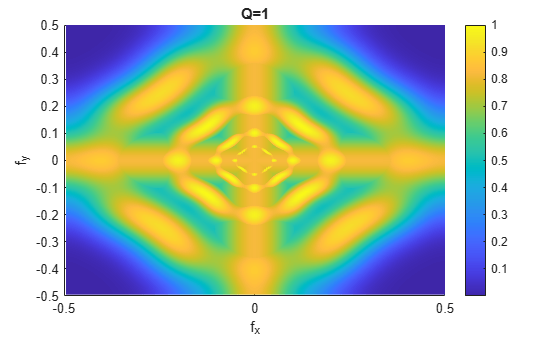littlewoodPaleySum

Littlewood-Paley sum

Description

lpsum = littlewoodPaleySum(sf) returns the Littlewood-Paley sum for the 2-D filter banks in the 2-D wavelet scattering network sf. lpsum is an M-by-N-by-Nfb matrix, where M-by-N is the matrix size of the padded filters and Nfb is the number of filter banks.

Since the scattering transform is contractive, the Littlewood-Paley sums do not exceed 1.

lpsum = littlewoodPaleySum(sf,fb) returns the Littlewood-Paley sum for the specified filter banks fb. fb is a positive integer or vector of positive integers between 1 and numfilterbanks(sf) inclusive. lpsum is an M-by-N-by-L matrix, where L is the number of unique elements in fb.

example

[lpsum,f] = littlewoodPaleySum(___) returns the spatial frequencies for the Littlewood-Paley sum. f is a two-column matrix with the first column containing the spatial frequencies in the x-direction and the second column containing the spatial frequencies in the y-direction.

Examples

collapse all

This example shows how to obtain and display the Littlewood-Paley sum of an image scattering network.

Create a scattering network with two filter banks and quality factors of 2 and 1, respectively.

sf = waveletScattering2('QualityFactors',[2 1]);

Obtain the Littlewood-Paley sums and spatial frequencies of the two filter banks. Display the maximum value of the sums. Since the scattering transform is contractive, the sums do not exceed 1.

[lpsum,f] = littlewoodPaleySum(sf);
max(max(lpsum(:,:,1)))
ans = 1.0000
max(max(lpsum(:,:,2)))
ans = 1.0000

Display the Littlewood-Paley sum of the second filter bank with the zero frequency centered. Note the 2-D Morlet filter bank used in the scattering transform is not designed to capture the highest spatial frequencies jointly in the x- and y-directions.

f(f>1/2) = f(f>1/2)-1;
surf(fftshift(f(:,1)),fftshift(f(:,2)),fftshift(lpsum(:,:,2)))
view(0,90)
xlabel('f_x')
ylabel('f_y')
colorbar
title('Q=1')Input Arguments

collapse all

Wavelet image scattering network, specified as a waveletScattering2 object.

Filter bank index in the image scattering network, specified as a positive integer or vector of positive integers between 1 and numfilterbanks(sf) inclusive. The number of filter banks in sf is equal to the number of specified QualityFactors in sf.

Output Arguments

collapse all

Littlewood-Paley sum for the filter banks in the image scattering network sf, returned as a real-valued 3-D matrix. lpsum is an M-by-N-by-L matrix, where M-by-N is the matrix size of the padded filters and L does not exceed the number of filter banks in sf.

Frequencies for the Littlewood-Paley sum, returned as a real-valued two-column matrix. Frequencies are in cycles per pixel. The first column of f contains the spatial frequencies in the x-direction, and the second column contains the spatial frequencies in the y-direction. In this convention, the Fourier transform is 1-periodic in both Fourier variables.